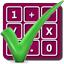#### Arithmetic of Computers

#### from Tenscope Limited

By using this site, you are accepting "session" cookies, as set out in the site's Privacy Policy
Cookies are also used to remember which page of the book you last viewed, so that when you revisit the site you automatically return to the last page you visited.

### Self-Test Questions : Lesson 2

#### The Arithmetic of Powers

##### Multiplication by addition, and division by subtraction

1.
If we multiplied 42 by 41, of what number would the product be a power?

2.
What is the exponent of the product when two numbers which are powers of the same base are multiplied?

3.
What power of 3 is (32)(33)?

4.
The sum of the exponents is the total number of times the base is used as a .....?..... in the product?

5.
Is it true that (34)(32) = 38?

6.
Is it true that (65)(64) = 69?

7.
If you multiply b5 by b2, what is the product?

8.
What is the product of bm times bn?

9.
In
6 5)30,
which is the divisor, the quotient, the dividend?

10.
In the expression (45)/(9) = 5, which is the divisor? The dividend? The quotient?

11.
What is the exponent of the quotient when one power of a base is divided by another power of the same base?

12.
What is another way of saying (bm)/(bn)?

13.
What is the quotient of b5 is divided by b2?

14.
What is b × b × b × b divided by b × b?

15.
Using exponents, divide 32 by 8.

16.
Write (36)/(33) first as a power of 3; then as a regular number.

17.
If 84 is divided by 84, what power of 8 results?

18.
Show how 33 is divided by 33 in two ways.

19.
What is the value of b0?

20.
What is (43)(40)?

21.
Is this statement true of false? 60 = 40 = 80 = 1

22.
What is the result when you divide 53 by 50?

Go now to Lesson 3 on Page 92.